Mobile QR Code1. (School of Electronic and Electrical Engineering, Hongik University / Seoul, Korea {ron999, youngmin}@hongik.ac.kr)

Approximate computing, 4-2 compressor, Multiplier, Power consumption, Delay, Accuracy

## 1. Introduction

Advancing the energy efficiency of a digital circuit is vital for many modern applications. To improve energy efficiency, a circuit that can reduce both delay and power consumption is designed. In general, there is a trade-off between delay and power consumption, but approximate computing methodology can be applied to improve both delay and power consumption. Therefore, approximate circuit design has been studied for a long time to improve performance, but there is no clear answer about which approximate circuit design is the best for the multiplication. It depends on the specific requirement, and the relationship between accuracy and performance is not proportional.

Previous designs have shown good performance with low accuracy [1-4]. On the other hand, other designs offer higher accuracy but lower performance [5-7]. The Momeni design  provides significant improvement in performance but has a fundamental accuracy limitation because it gives non-zero results for zero inputs, which results in substantial relative errors for small operands used in the multiplication . Therefore, the accuracy needs to be improved.

In this study, a new approximate 4-2 compressor design is proposed to reduce the error rate of an approximate multiplier. It gives zero results for zero inputs. An approximate multiplier composed of the proposed compressor shows improved error metrics with less power consumption compared with a previous design . A high-performance 32-nm predictive technology model (PTM) simulation proves that the proposed 4-2 compressor design improves the performance compared with the other design . The proposed multiplier has lower power consumption and error rate than an approximate multiplier that uses the Momeni design . In addition, the proposed design is more accurate in small operand multiplication.

The rest of paper is organized as follows. Section 2 presents a review of exact and Momeni compressors  and introduces the proposed design of an approximate 4-2 compressor. The Dadda multiplier is explained, and multipliers using the proposed compressor are introduced in Section 3. Section 4 presents the implementation results and analysis of the transistor-level compressor and multiplier. Section 5 concludes the paper.

## 2. Previous 4-2 Compressor

The Momeni design  has non-zero results for zero inputs, resulting in large relative errors for small operands. The proposed design is presented to compensate for the weakness of the Momeni design  with improved performance. It was implemented at a transistor level and investigated through a probability analysis.

### 2.1 Exact 4-2 Compressor

Since an exact 4-2 compressor has five inputs (denoted as x1, x2, x3, x4, C_IN) and three outputs (SUM, CARRY, C_OUT), it is called a (5,3) counter. In Fig. 1, SUM has the same weight as the inputs, while CARRY and C_OUT have double the weight. C_OUT is independent of C_ IN. For instance, C_OUT of column i column i becomes C_IN of column i+1, and this feature is used in tree multipliers. The usual implementation of an exact 4-2 compressor is represented by using two full adders .

##### Fig. 1. An exact 4-2 compressor with two full adders.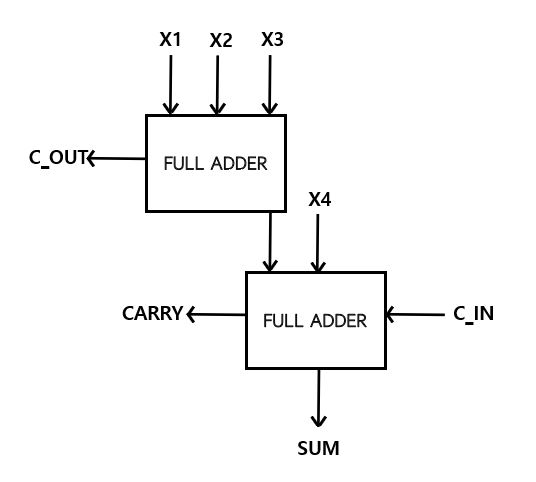### 2.2 Momeni Approximate 4-2 Compressor

A Momeni approximate 4-2 compressor starts with a truth table. CARRY from the truth table of an exact 4-2 compressor has the same value with the C_IN, 24 out of 32 states. Therefore, CARRY is simplified to C_IN to design an approximate 4-2 compressor. The SUM and C_OUT output equation is simplified to reduce the complexity of the circuit. The expressions are described below.

##### (1)
$Carry=C_{-}IN \\$
##### (2)
$Sum=\overline{C_{-}IN}\left(\overline{X1\oplus X2}+\overline{X3\oplus X4}\right) \\$
##### (3)
$C_{-}OUT=(\overline{\overline{X1X2}+\overline{X3X4}})$

If C_OUT is always equal to C_IN, C_OUT and C_IN can be ignored in the circuit to design a simpler one, so we replace C_OUT on the left side of Eq. (3) with CARRY on the left side of Eq. (1). Then, the output equation can be more straightforward, and the circuit design is simpler. Therefore, the critical path delay and power consumption are expected to improve compared with an exact 4-2 compressor. The result of the Momeni design  is shown in Table 1. Fig. 2 shows the gate-level implementation of the Momeni approximate 4-2 compressor, and output equations are described below.

##### (4)
$SUM=\left(\overline{X1\oplus X2}+\overline{X3\oplus X4}\right) \\$
##### (5)
$CARRY=(\overline{\overline{X1X2}+\overline{X3X4}})$

In Table 1, the design has four incorrect results out of 16 outputs, so its error rate is 25%, but it has a significant defect. Because it has an incorrect result at x4x3x2x1=0000, the probability of this order is 0.32, which is higher than the others. This will make significant relative errors for small operand operations when the Momeni compressor is used in the multiplier.

##### Table 1. Truth table of the Momeni compressor.
 X4 X3 X2 X1 CARRY SUM Difference 0 0 0 0 0 1 +1 0 0 0 1 0 1 0 0 0 1 0 0 1 0 0 0 1 1 0 1 -1 0 1 0 0 0 1 0 0 1 0 1 1 0 0 0 1 1 0 1 0 0 0 1 1 1 1 1 0 1 0 0 0 0 1 0 1 0 0 1 1 0 0 1 0 1 0 1 0 0 1 0 1 1 1 1 0 1 1 0 0 0 1 -1 1 1 0 1 1 1 0 1 1 1 0 1 1 0 1 1 1 1 1 1 -1
##### Fig. 2. Gate-level implementation of Momeni approximate 4-2 compressor.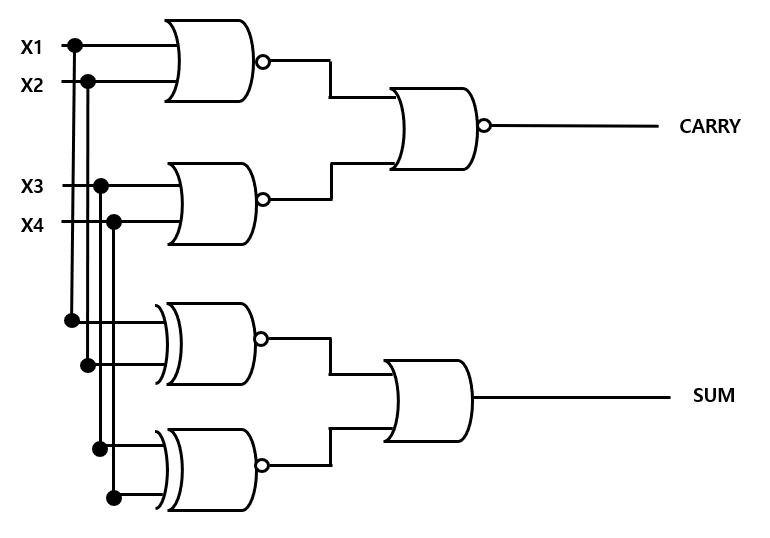### 2.3 Proposed 4-2 Compressor

The proposed 4-2 compressor is introduced to improve the error rate, the delay, and the power consumption. In order to improve the performance of the compressor, the importance of the input order should be analyzed and understood first. The partial product from the AND gate has the same probability of being 1. The probability is 1/4. This partial product becomes the input of the 4-2 compressor in the multiplier, so the probability of x4x3x2x1 = 0001 and the probability of x4x3x2x1 = 0111 are different.

For example, the probability of x4x3x2x1 = 0001 is 27/256, and that of x4x3x2x1 = 0111 is 3/256. If x4x3x2x1 = 0001 and x4x3x2x1 = 0111 are the error order input of approximate 4-2 compressor, the x4x3x2x1 = 0001 order will make more error than x4x3x2x1 = 0111 order due to the probability in the multiplier. Therefore, an input order that makes an error result, like x4x3x2x1 = 0000 and x4x3x2x1 = 0001, is not recommended. Instead, the input orders x4x3x2x1 = 0011 and x4x3x2x1 = 0111 are recommended. To improve the performance of the approximate 4-2 compressor, we assume C_ IN and C_OUT of the proposed design have the same weight as in the Momeni design .

First, making the correct output is needed when the input ordering is x4x3x2x1 = 0000, x4x3x2x1 = 0001, x4x3x2x1 = 0010, x4x3x2x1 = 0100, and x4x3x2x1 = 1000. The AND of x1 and x2 can make correct CARRY outputs from the order of inputs with high probability, but it makes eight incorrect outputs. Eight incorrect outputs are reduced to five when combined with the AND of x3 and x4 using OR gates. Using De Morgan’s laws, the CARRY equation uses only NAND, and the following describes the CARRY output.

##### (6)
$\begin{equation} SUM=\overline{\left(\overline{X1\oplus X2}\right)\left(\overline{X3+X4}\right)} \end{equation}$

SUM has to make the correct outputs when the input order has a high probability. The XOR of x1 and x2 produces the correct result except when the input order is x4x3x2x1 = 0100. However, The OR of x3 and x4 has the correct result when combined with the XOR of x1 and x2 using an OR gate. Using De Morgan's laws, the SUM equation uses NAND and NOR to improve the delay and power consumption, and we describe the CARRY output below. Fig. 3 shows the gate-level implementation of the proposed approximate 4-2 compressor.

##### (7)
$\begin{equation} CARRY=\overline{\left(\overline{X1X2}\right)\left(\overline{X3X4}\right)} \end{equation}$

Table 2 shows the truth table of the proposed approximate 4-2 compressor. There are no incorrect outputs when the input ordering is x4x3x2x1 = 0000, x4x3x2x1 = 0001, x4x3x2x1 = 0010, x4x3x2x1 = 0100, and x4x3x2x1 = 1000, and the proposed compressor has six incorrect outputs out of 16 outputs. It will make fewer errors for small operand operations when the proposed compressor is used in a multiplier. Fig. 3 shows that the number of gates used is reduced compared with the Momeni design  because a NOR gate is used in the SUM equation of the proposed design instead of an XNOR gate. Therefore, so the proposed compressor will show improvement in the power consumption and delay.

##### Fig. 3. Gate-level implementation of proposed approximate 4-2 compressor.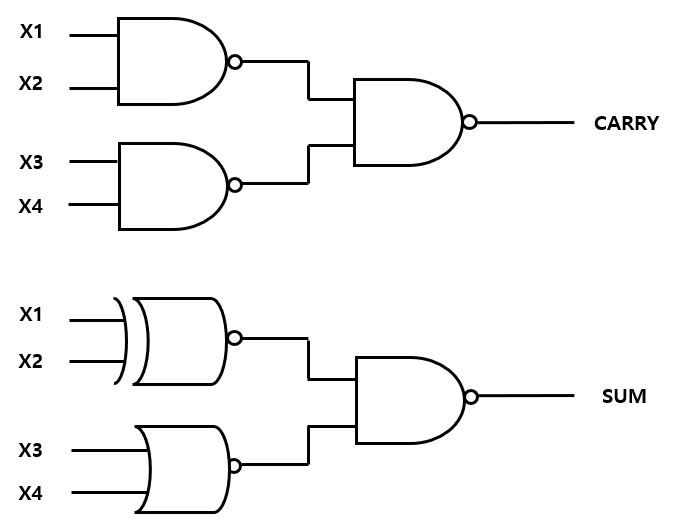##### Table 2. Truth table of the proposed compressor.
 X4 X3 X2 X1 CARRY SUM Difference 0 0 0 0 0 0 0 0 0 0 1 0 1 0 0 0 1 0 0 1 0 0 0 1 1 1 0 0 0 1 0 0 0 1 0 0 1 0 1 0 1 -1 0 1 1 0 0 1 -1 0 1 1 1 1 1 0 1 0 0 0 0 1 0 1 0 0 1 0 1 -1 1 0 1 0 0 1 -1 1 0 1 1 1 1 0 1 1 0 0 1 1 +1 1 1 0 1 1 1 0 1 1 1 0 1 1 0 1 1 1 1 1 1 -1

## 3. Multiplication

The impact of the proposed compressor for multiplication was investigated using an 8${\times}$8 unsigned Dadda multiplier. In general, a multiplier is composed of three parts :

· Partial Product Generation (PPG)

· Partial Product Summation Trees (PPST)

The second stage, PPST, has a dominant role in delay, power consumption, and circuit complexity. Since the approximation can reduce circuit complexity, it has suitable characteristics to be applied to this stage. Therefore, approximate compressors have been widely used to reduce the delay and power dissipation. In the PPG stage, an AND gate generates all partial products. Approximate compressors are used to reduce the partial product in the PPST stage.

In the FA stage, a 15-bit full adder is used. The upper bit of the 8${\times}$8 unsigned Dadda multiplier plays a significant role in the result of multiplication. Therefore, the proposed approximate compressor is applied up to 7 bits in the PPST stage to reduce the difference between an exact value and approximate value. Fig. 4 shows the reduction circuit of an 8${\times}$8 Dadda multiplier. The following three multipliers were designed and compared:

##### Fig. 4. 8×8 Dadda multiplier: (a) using 4-2 compressor for all bits; (b) using approximate 4-2 compressor in low 7bits.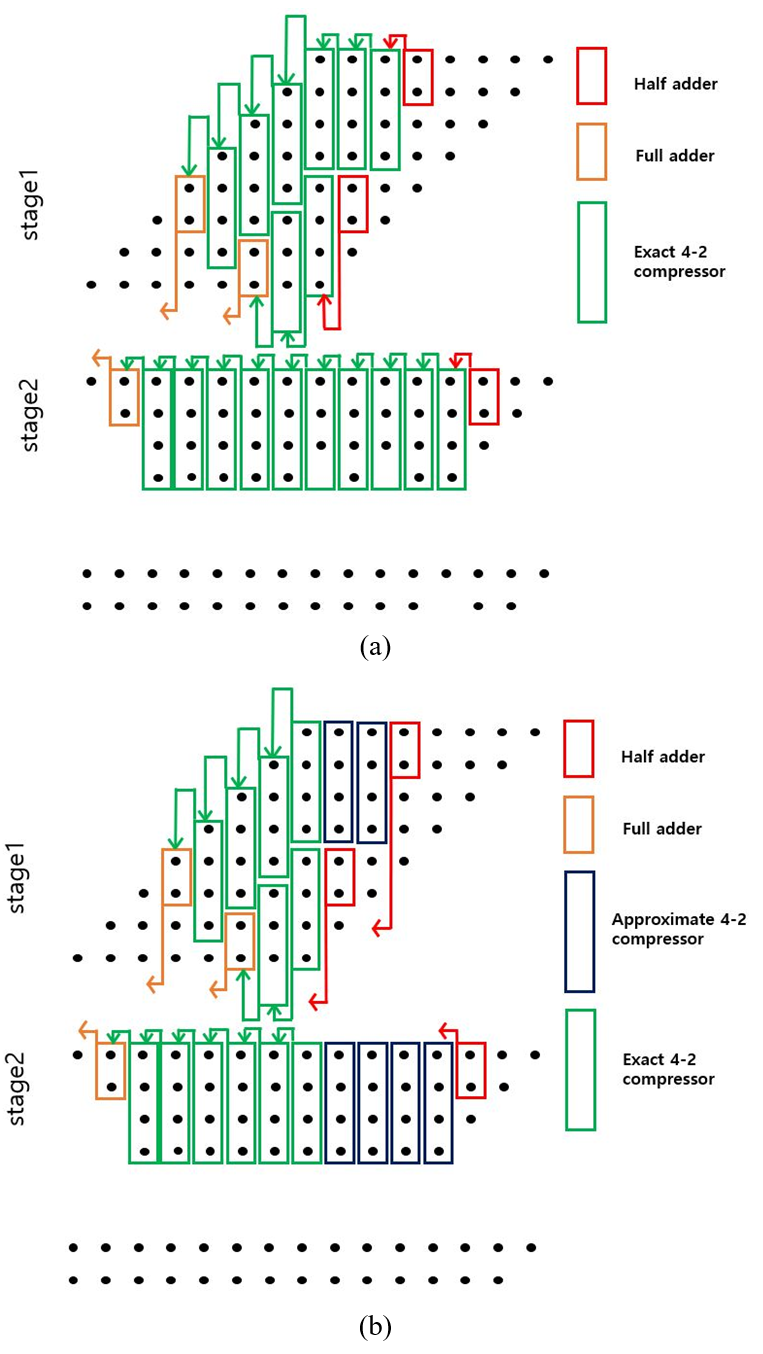· Multiplier 1 (MUL1): Exact 4-2 compressors  are used in Fig. 4(a)

· Multiplier 2 (MUL2): The Momeni design  is used for all approximate 4-2 compressors in Fig. 4(b).

· Proposed multiplier: The proposed approximate 4-2 design is used for all approximate 4-2 compressors in Fig. 4(b).

The main objective of MUL2 and the proposed multiplier is to reduce power consumption compared with MUL1 because they are approximate computations. The exact compressors determine the delay in the MUL2 and proposed designs in the critical path, so there is no significant delay improvement in MUL2 and the proposed compared with MUL1.

### 3.1 Error Rate Minimization

In the first stage, the 4-2 compressor inputs are partial products and independent of each other. The probability of being 1 is 1/4, but inputs of the second stage for 4-2 compressors come from the first stage, so the approximate 4-2 compressor inputs are dependent, as shown in Figs. 5 and 6. Before understanding the relationship between the input ordering of the approximate 4-2 compressor and the approximate multiplier's error rate, calculating the probability of the output from the first stage is needed.

The probability that the sum of half adder is 1 is: P(sum) = p(x1x2 = 01) + p(x1x2 = 10) = (3/4)(1/4) + (1/4)(3/4) = 0.375. The probability that carry becomes 1 is: P(carry) = p(x1x2 = 11) = (1/4)(1/4) = 0.062. In the Momeni design, the probability that sum is 1 is: P(sum) = 1 – p(x4x3x2x1 = 0101) – p(x4x3x2x1 = 0110) – p(x4x3x2x1 = 1001) – p(x4x3x2x1 = 1010) = 0.859. The other probabilities are also calculated in this way. P(carry) of the Momeni design being 1 is 0.191. In the proposed design, the probability that sum is 1 is P(sum) = 0.648, and the probability that carry is 1 is P(carry) = 0.168.

To minimize the error rate of an approximate multiplier, an input order that makes the minimal probability of an error result in the second stage of the PPST is needed. In the Momeni compressor, the orders x4x3x2x1 = 0000, x4x3x2x1 = 0011, x4x3x2x1 = 1100, and x4x3x2x1 = 1111 show error results. The probability of the orders of x4x3x2x1 = 0000 and x4x3x2x1 = 1111 is independent of the input order, but the probability of the orders x4x3x2x1 = 0011 and x4x3x2x1 = 1100 has an input order dependency.

At 5 bits, three partial products have the same probability, so the sum of the half adder from the first stage does not affect the result. P(sum) of the Momeni design is relatively high at 6 bits and 7 bits in Fig 5. Therefore, P(sum) has to pair up with the input with the lowest probability at those bits in x1x2 or x3x4. The input order that makes a minimal error result is shown in Fig. 5.

##### Fig. 5. Partial product probability of the second stage of the multiplier ofFig. 4(b)using Momeni design. This shows the input ordering that minimizes the error probability for MUL2 in the second stage.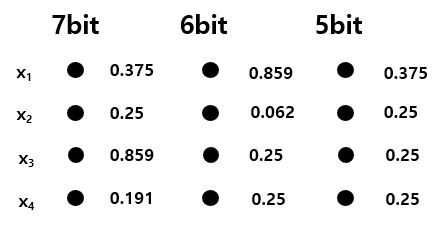In the proposed compressor, the orders x4x3x2x1 = 0101, x4x3x2x1 = 0110, x4x3x2x1 = 1001, x4x3x2x1 = 1010, x4x3x2x1 = 1100, and x4x3x2x1 = 1111 show error results. x4 and x3 make an error four times when x4 and x3 values are 1. x1 and x2 make an error three times when x1 and x2 values are 1. To minimize the error probability, the first and second lowest probabilities should have an input of x4 and x3. The input orders that make a minimal error result are shown in Fig. 6. The objective of the proposed design is to reduce the error rate compared with MUL2. Input order x4x3x2x1 = 0000 of the Momeni approximate compressor used in MUL2 will make significant relative errors for small operand operations, but the proposed approximate compressor has a correct result at x4x3x2x1 = 0000 and will show a better error rate than MUL2.

##### Fig. 6. Partial products probability of the second stage of the multiplier of Fig. 4(b) using the proposed design. This figure shows the input ordering that minimizes the error probability for the proposed multiplier in the second stage.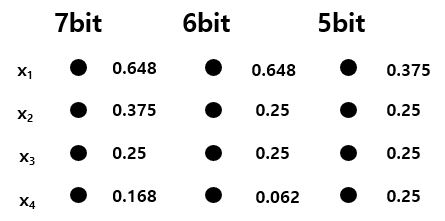## 4. Simulation Results

This section explains the simulations of the three 4-2 compressor designs and three 8${\times}$8 unsigned Dadda multipliers using HSPICE. 32-nm high-performance PTM model is utilized in a transistor-level simulation. The settings used are channel length = 30 nm, Wn = 60 nm, Wp = 120 nm, and power supply VDD = 1.0 V. The results are summarized in Tables 3 and 4.

### 4.1 Approximate Compressor

One exact compressor and two approximate compressors were simulated. The simulation results of the delay, power consumption, and power-delay product (PDP) are summarized in Table 3. As shown in Table 3, the proposed design has the best delay, power consumption, and PDP. The proposed approximate compressor is 81.71% faster than the exact compressor and 22.51% faster than Momeni approximate compressor. The power consumption shows 63.2% improvement compared to the exact compressor and 4.24% reduction compared to the Momeni approximate compressor. The speedup and decrease in power consumption in the proposed compressor are attributed to reducing the number of transistors. The proposed approximate compressor shows a 61.91% reduction from the exact compressor and a 23.81% reduction compared to the Momeni approximate compressor.

##### Table 3. Simulation results of compressor (32 nm).
 Design Delay Power PDP # of transistors (ps) normalized (uW) normalized (aJ) normalized (ea) normalized Exact  74.35 5.47 9.81 2.72 729.37 14.85 84 2.63 Momeni  17.55 1.29 3.77 1.04 66.16 1.35 42 1.31 Proposed 13.60 1.00 3.61 1.00 49.1 1.00 32 1.00

### 4.2 Approximate Multiplier

Three multipliers were simulated: exact, MUL2, and the multiplier with proposed compressors, and the results are summarized in Table 4. As shown in Table 4, the proposed design has the best delay, power consumption, and PDP. In Table 4, there is no significant delay improvement in the proposed multiplier compared with the exact and MUL2 multipliers because the critical paths among all three multipliers are the same. However, the proposed multiplier’s power consumption shows an 18.65% improvement compared to the exact multiplier and a 4.18% reduction compared to the approximate MUL2. The decrease in power consumption is attributed to reducing the transistors in the PPST stage. The proposed method shows a 13.44% reduction compared to MUL1 and a 2.9% reduction compared to MUL2 in terms of the number of transistors.

##### Table 4. Simulation results of multiplier (32 nm).
 Design Delay Power PDP # of transistors (ps) normalized (uW) normalized (aJ) normalized (ea) normalized MUL1  154.00 1.01 253.59 1.30 390.53 1.24 2322 1.16 MUL2  153.00 1.00 215.30 1.04 329.41 1.04 2070 1.03 Proposed 153.00 1.00 206.30 1.00 315.64 1.00 2010 1.00

### 4.3 Error Metrics

MUL2 and the proposed multiplier were simulated to compare the error metrics for an 8${\times}$8 unsigned Dadda approximate multiplier. The Error Rate (ER), Mean Error Distance (MED), and Normalized Mean Error Distance (NMED) are summarized in Tables 5 and 6. Assuming E and A, the results were produced by an exact and an approximate multiplier. First, we define the Error Distance , ED = ${\sum}$|E-A|, and the Max Out for unsigned multiplier, Max Out = (2$^{\mathrm{n}}$-1)$^{2}$. The error metrics considered in this paper are:

· Error Rate (ER): the percentage of the multiplication result for which ED > 0.

· Mean Error Distance (MED): ED/2$^{\mathrm{2n}}$ = ${\sum}|E-A|/2$$^{2n}$.

· Normalized Error Distance (NMED): the average value of ED divided by Max Out.

As shown in Table 7, the proposed multiplier shows better accuracy than MUL2. As mentioned, MUL2 shows a 93.43% error rate because incorrect order x4x3x2x1 = 0000 of the Momeni approximate compressor results in significant relative errors for small operand operation. However, the proposed design shows a 15.54% improvement in ER compared with MUL2 because the input order x4x3x2x1 = 0000 of the proposed approximate design results in an exact value of 0. The proposed multiplier also provides better error performance in MED and NMED than MUL2.

To prove the robustness of the proposed design for multiplication between small operands compared with the Momeni method (i.e., MUL2), multiplication results from 0${\times}$0 to 63${\times}$63 were investigated and are summarized in Table 8. It is worth noting that the improvement compared with MUL2 becomes larger for smaller operands. From this result, the multiplier using the Momeni compressor is more susceptible to multiplication between small operands, but the multiplier with the proposed compressor is not.

##### Table 5. Error Performance of 8${\times}$8 unsigned approximate multipliers from 0 - 255 (2$^{8}$-1).
 ER (%) MED NMED MUL2 93.43 167.21 0.026 Proposed 77.89 157.21 0.024 Improvement w.r.t. MUL2 15.54% 5.98% 7.69%
##### Table 6. Error performance of 8${\times}$8 unsigned approximate multipliers from 0 - 63 (2$^{6}$-1).
 ER (%) MED NMED MUL2 92.19 33.24 0.51×10$^{-3}$ Proposed 69.87 28.46 0.43×10$^{-3}$ Improvement w.r.t. MUL2 22.32% 14.38% 15.69%

## 5. Conclusion

This paper introduced a new approximate 4-2 compressor design and a multiplier using the proposed compressor. The proposed designs were compared with previous compressors and multipliers. Based on the analysis, the following conclusions can be drawn.

· Reducing the number of the transistors can improve both power consumption and delay.

· The approximate compressor error rate from the truth table is not the only one factor that determines the error rate of the multiplier. Instead, the probability of the input order that makes the error result needs to be considered.

· Approximate compressors with error order generating a non-zero result for zero inputs  are susceptible to small operands. However, the proposed multiplier design is stronger for small operands because it was designed based on the probability to minimize errors for small operand multiplication.

The proposed compressor and multiplier show better performance than the Momeni design  and are strong for small operands.

### REFERENCES

1
Momeni A., Han J., Montuschi P., Lombardi F., April 2015, Design and Analysis of Approximate Compressors for Multiplication, in IEEE Transactions on Computers, Vol. 64, No. 4, pp. 984-9942
Sabetzadeh F., Moaiyeri M. H., Ahmadinejad M., Nov. 2019, A Majority-Based Imprecise Multiplier for Ultra-Efficient Approximate Image Multiplication, in IEEE Transactions on Circuits and Systems I: Regular Papers, Vol. 66, No. 11, pp. 4200-42083
Ahmadinejad M., Moaiyeri M. H., Sabetzadeh F., Oct. 2019., Energy and area efficient imprecise compressors for approximate multiplication at nanoscale, AEU - International Journal of Electronics and Communications, Vol. 1104
Akbari O., Kamal M., Afzali-Kusha A., Pedram M., April 2017, Dual-Quality 4:2 Compressors for Utilizing in Dynamic Accuracy Configurable Multipliers, in IEEE Transactions on Very Large Scale Integration (VLSI) Systems, Vol. 25, No. 4, pp. 1352-13615
Yang Z., Han J., Lombardi F., 2015, Approximate compressors for error-resilient multiplier design, 2015 IEEE International Symposium on Defect and Fault Tolerance in VLSI and Nanotechnology Systems (DFTS), pp. 183-1866
Lin C., Lin I., 2013, High accuracy approximate multiplier with error correction, 2013 IEEE 31st International Conference on Computer Design (ICCD), pp. 33-387
Ha M., Lee S., March 2018, Multipliers With Approximate 4-2 Compressors and Error Recovery Modules, in IEEE Embedded Systems Letters, Vol. 10, No. 1, pp. 6-98
Strollo A. G. M., Napoli E., De Caro D., Petra N., Meo G. D., Sept. 2020, Comparison and Extension of Approximate 4-2 Compressors for Low-Power Approximate Multipliers, in IEEE Transactions on Circuits and Systems I: Regular Papers, Vol. 67, No. 9, pp. 3021-30349
Edavoor P. J., Raveendran S., Rahulkar A. D., 2020, Approximate Multiplier Design Using Novel Dual-Stage 4:2 Compressors, in IEEE Access, Vol. 8, pp. 48337-4835110
Roy A. S., Dhar A. S., 2018, A Novel Approach for Fast and Accurate Mean Error Distance Computation in Approximate Adders, 2018 IEEE International Symposium on Circuits and Systems (ISCAS), pp. 1-5## Author

Jahyung Gu is in the BAc program at the IC Design & Embedded-system Application Laboratory (IDEA LAB) for electronic and electrical engi-neering at Hongik University, Seoul, Korea. He received a BSc in electronic and electrical engineering from Hongik University, Seoul, Korea, in 2022. His research interests include embedded systems, design and technology co-optimization methodologies, and low-power and 3D IC designs.

Youngmin Kim received a BSc in electrical engineering from Yonsei University, Seoul, Korea, in 1999 and an MSc and a PhD in electrical engineering from the University of Michigan, Ann Arbor, in 2003 and 2007, respectively. He held a senior engineering position at Qualcomm in San Diego, CA. He is currently a Full Professor at Hongik University, Seoul, South Korea. Prior to joining Hongik University, he was with the School of Computer and Information Engineering at Kwangwoon University, Seoul, South Korea, and the School of Electrical and Computer Engineering at the Ulsan National Institute of Science and Technology (UNIST), Ulsan, South Korea. His research interests include embedded systems, variability-aware design methodologies, design for manufacturability, design and technology co-optimization methodologies, and low-power and 3D IC designs.